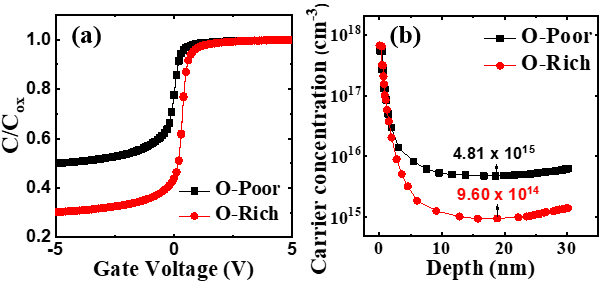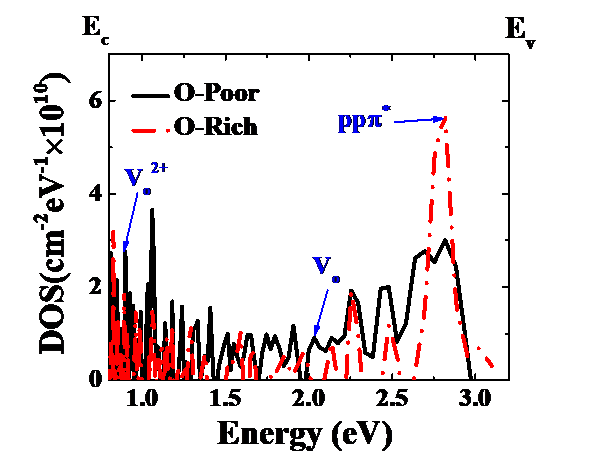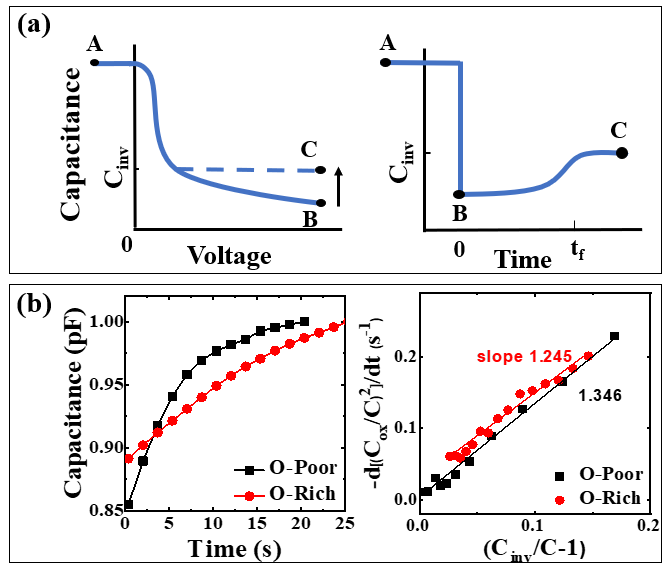Mobile QR Codea-IGZO TFT, channel defect, DOS, c-t, carrier lifetime

## I. INTRODUCTION

##### (2)
$$\mu_{F E}=\frac{g_{m}}{C_{o x} \times W / L^{\times V_{D}}}$$

Similar to the results of previous papers, $\mathrm{V}_{\mathrm{th}}$ shifted positively as the OFR increased, and the $\mu_{F E}$, SS, and current ratio ($\mathrm{I}_{\mathrm{on}} / \mathrm{I}_{\mathrm{O} \mathrm{ff}}$) did not change significantly (3). The reason for the $\mathrm{V}_{\mathrm{th}}$ positive shift is that as the OFR increases, more oxygen vacancies are compensated for by the injected oxygen and the conductivity of the channel is lowered, leading to a decrease in carrier concentration.

Table 1. Electrical parameters of a-IGZO TFTs with different OFRs.

 OFR $\mathrm{V}_{\mathrm{th}}$ (V) $\mu_{\mathrm{FE}}\left(\mathrm{cm}^{2} / \mathrm{V} \mathrm{s}\right)$ SS (V/dec) $\mathrm{I}_{\mathrm{on}} / \mathrm{I}_{\mathrm{O} \mathrm{ff}}$ O-Poor -0.22 7.5 0.24 1.50×108 O-Rich 0.85 7.5 0.25 1.52×108

Fig. 3 shows the carrier concentration according to the C-V measurement results of the MOS capacitor and the depth extracted from the C-V data. Through the C-V measurement results, it was confirmed that the higher the OFR, the more that $\mathrm{V}_{\mathrm{FB}}$ shifts positively in accordance with the TFT's $\mathrm{V}_{\mathrm{th}}$ shift trend. The inversion capacitance

Fig. 3. (a) C-V plot of the MOS capacitors, (b) Carrier concentration according to IGZO depth (nm).of the O-poor rate was increased because the carrier concentration of a-IGZO was increased. The total capacitance of the MOS capacitor is determined based on the electron capacitance ($\mathrm{C}_{\mathrm{n}}$), hole capacitance ($\mathrm{C}_{\mathrm{p}}$), interface trap capacitance ($\mathrm{C}_{\mathrm{it}}$), and space charge region bulk capacitance ($\mathrm{C}_{\mathrm{b}}$). However, under the inversion bias condition, electrons move away from the surface, where holes and interface traps are only present in small amounts that do not respond at high frequency, so the effects of $\mathrm{C}_{\mathrm{n}}$, $\mathrm{C}_{\mathrm{p}}$, and $\mathrm{C}_{\mathrm{it}}$ are ignored. Therefore, the influences of $\mathrm{C}_{\mathrm{ox}}$ and $\mathrm{C}_{\mathrm{b}}$ are dominant relative to the total capacitance and can be expressed as Eq. (3)(7):

##### (3)
$$\begin{array}{l} C_{\text {total}}=\frac{1}{1 / C_{\text {ox}}+1 /\left(C_{n}+C_{p}+C_{i t}+C_{b}\right)} \approx \frac{C_{\alpha x} C_{b}}{C_{\alpha x}+C_{b}} \\ C_{b}=\frac{\kappa_{s} \varepsilon_{0}}{W_{i n v}} \end{array}$$

where K$_{\mathrm{s}}$ is the semiconductor dielectric constant, $\varepsilon_{0}$ is the permittivity of free space, and $\mathrm{W}_{{inv }}$ is the inversion space-charge region width. $\mathrm{W}_{{inv }}$ decreases due to the electrons originating from the increased oxygen vacancy of the O-poor. Fig. 3(b) shows that the carrier concentration increased with a lower OFR. It can be seen that as the oxygen supply decreases, the oxygen vacancy increases, and thus the carrier concentration increases.

Fig. 4 shows the PECCS measurement results of TFTs with different OFRs. The neutral, ionized oxygen vacancy and metastable ($p p \pi^{*}$) defects of IGZO exist in the E$_{\mathrm{v}}$ + 1.1 eV, E$_{\mathrm{c}}$ - 0.05 eV, and E$_{\mathrm{v}}$ + 0.15 eV energy level states (1). It was confirmed that as the OFR increased, the oxygen vacancy decreased and the O-O bond increased. The DOS extracted through PECCS was identical to previously reported XPS results (4). Through these results, it is possible to identify the cause of the $\mathrm{V}_{\mathrm{th}}$ positive shift of the O-rich TFTs in the $\mathrm{I}_{\mathrm{D}}-\mathrm{V}_{\mathrm{G}}$ plot and the

Fig. 4. DOS in IGZO TFTs, extracted by photoexcited charge collection spectroscopy.Fig. 5. (a) C-V and c-t behavior of bias applied to MOS capacitors by deep depletion, (b) Zerbst plot.increase of the inversion capacitance value of the O-poor in the C-V curve.

Fig. 5(a) shows the C-V and c-t behavior in the deep depletion state of a bias (±5 V) applied MOS capacitor. The generation lifetime measurement taken using the c-t method relies on the fact that the IGZO channel reaches the deep depletion state when a momentary reversal voltage is applied. Deep depletion is formed in the channel region when the device is switched to the inversion voltage momentarily after the accumulation voltage is applied. Over time, electron hole pairs (EHP) generation occurs from impurities in the IGZO (oxygen vacancy defect), and as the number of majority carriers charged in the capacitor increases, the reverse capacitance (C$_{\mathrm{inv}}$) gradually recovers, leading to state C. The recovery time (t$_{\mathrm{f}}$) in the c-t plot is determined by the amount of EHP in IGZO. The recovery time of the O-poor TFT appeared short, which means that more defects are distributed in the channel region. As such the generation lifetime ($\tau_{g}$), defined as Eq. (4), can be measured via c-t characterization (7):

##### (4)
$$\tau_{g}=\frac{2 n_{i} C_{o x}}{N_{A} C_{i n v} \text { slope }}$$

where n$_{\mathrm{i}}$ is the IGZO intrinsic carrier density (1.71 x 102 cm-3) (2), N$_{\mathrm{A}}$ is the acceptor doping density, and S is the slope in the -d(C$_{\mathrm{ox}}$/C)$^{2}$/dt-(C$_{\mathrm{inv}}$/C)$^{-1}$ plot. Fig. 5(b) shows the results of the c-t measurements and Zerbst plot extraction. The $\tau_{g}$ extracted through this process was higher with an increasing OFR, the $\tau_{g}$ of the O-poor sample was 5.86 ${\times}$ 10$^{-14}$ (s), and the $\tau_{g}$ of the O-rich sample was 5.54 ${\times}$ 10$^{-13}$ (s).

## V. CONCLUSIONS

We proposed a method to identify the channel characteristics of IGZO TFTs through electrical analysis without physical analysis. By fabricating devices with different OFRs that have been reported previously, the interface characteristics of the IGZO and gate insulator were analyzed through PECCS to compare the number of defects according to the energy level. Bulk IGZO was investigated by extracting the carrier lifetime through c-t measurements. Finally, by verifying the same results as previously reported through physical analysis, the effectiveness of the electrical analysis method presented in this paper was confirmed. In future studies, the quality index can be affirmed when IGZO film quality is improved using the electrical property evaluation presented in this paper.

### ACKNOWLEDGMENTS

This work was supported by the National Research Foundation of Korea (NRF) grant funded by the Korea government (MSIT) (No. 2019R1F1A1051493). And, this research was funded and conducted under ‘the Competency Development Program for Industry Specialists’ of the Korean Ministry of Trade, Industry and Energy (MOTIE), operated by Korea Institute for Advancement of Technology (KIAT) (No. P0012453, Next-generation Display Expert Training Project for Innovation Process and Equipment, Materials Engineers).

### REFERENCES

1
Jang J. T., Feb., 2018, Effects of structure and oxygen flow rate on the photo-response of amorphous IGZO-based photodetector devices, Solid-State Electronics, Vol. 140, No. , pp. 115-1212
Kim Y. S., Oct., 2012, Amorphous InGaZnO Thin-Film Transistors-Part I: Complete Extraction of Density of States Over the Full Subband-Gap Energy Range, IEEE Trans on Electron Device, Vol. 59, No. 10, pp. 2689-26983
Kim S. C., Jan., 2012, Impact of Oxygen Flow Rate on the Instability Under Positive Bias Stresses in DC-Sputtered Amorphous InGaZnO, IEEE Electron Device Letter, Vol. 33, No. 1, pp. 62-644
Yoon J. S., Sep., 2018, Hybrid complementary inverter based on carbon nanotube and IGZO thin-film transistors with controlled process conditions, Journal of Alloys and Compounds, Vol. 762, No. 25, pp. 456-4625
Eric kai-Hsiang Yu , Mar., 2018, photoluminescence study of Amorphous InGaZnO Thin-Film Transistors, IEEE Trans on Electron Device, Vol. 65, No. 3, pp. 1258-12616
Kim H. J., May, 2020, The Effects of Valence Band Offset on Threshold Voltage Shift in a-InGaZnO TFTs Under Negative Bias Illumination Stress, IEEE Electron Device Letter, Vol. 41, No. 5, pp. 737-7407
Schroder D. K., 2006, Semiconductor Material and Device Characterization, 3rd ed., Wiley, New York## Author

Hyo-Jung Kim received his M.S. degree in the Department of Infor-mation and Communication Engi-neering from Sungkyunkwan Univer-sity, Suwon, South Korea, in 2013.

He is currently a Ph.D. candidate with the Department of Semiconductor and Display Engineering in Sungkyunkwan University, Suwon, South Korea.

In 2013, he joined the Samsung Display Company where he has been working in the area of quality and reliability.

His interests are the reliability of Oxide TFTs and LTPS for flexible displays.

Soon-Kon Kim received his B.S. and M.S. degrees in the Department of Information and Communication Engineering, Sungkyunkwan Univer-sity, Suwon, South Korea., in 2013 and 2015, respectively.

He is currently a Ph.D. candidate in Sungkyunkwan University, Suwon, south Korea.

His current research interests include display devices.

Jung-Min Park received his B.S. degree in Materials science engi-neering from Korea University, Seoul, South Korea, in 2002 and his M.S. degree in Materials Science Engineering from the Gwangju Institute of Science and Technology, Gwangju, South Korea, in 2004.

He is currently a Ph.D. candidate with the Department of Semiconductor and Display Engineering in Sungkyunkwan University, Suwon, South Korea.

Since 2004, he has been with the Samsung Electronics Company, Ltd., Gyeonggi-Do, South Korea, and has been engaged in the development of high-k gate dielectric and metal gates for advanced logic devices.

Ki-Hwan Kim received his B.S. and M.S. degrees in the Department of Physics from Korea University, Seoul, south Korea, in 2004 and 2006, respectively.

He is currently a Ph.D. candidate with the Department of Semiconductor and Display Engineering in Sungkyunkwan University, Suwon, South Korea.

In 2006 he joined Hynix (now, SK-hynix) and in 2011 he joined the Samsung Display Company where he has been working in the area of computer aided engineering.

His interests include TCAD analysis and the reliability of oxide TFTs LTPS for displays.

Jang-Kun Song received his B.S. and M.S. degrees in physics from Korea University, Seoul, South Korea in 1994 and 1996, respectively.

He also received his Ph.D. degree in electronic engineering from the University of Dublin, Dublin, Ireland in 2008.

He worked in the R&D department of Samsung Electronics Company as a principal engineer from 1996 to 2010.

In Samsung, he invented the patterned vertical alignment LCD technology, which is currently the main technology used in LCD applications produced by the Samsung Company.

In 2010, he moved to Sungkyunkwan University, Suwon, South Korea, and he is currently an associate professor in the School of Electronics and Electrical Engineering.

Byoung-Deog Choi received the Ph.D. degree in electrical engi-neering from Arizona State Univer-sity, Tempe, AZ, USA, in 2002.

He is currently a Professor with the School of Information and Com-munication Engineering, Sungkyun-kwan University, Suwon, South Korea.

He has authored over 100 articles in SCI journals and holds 50 patents.

His current research interests include display devices and C-MOS technology.